# ISEE Middle Level Math : Numbers and Operations

## Example Questions

### Example Question #1 : Use Proportional Relationships To Solve Multistep Ratio And Percent Problems: Ccss.Math.Content.7.Rp.A.3

200 percent of what number is equal to the difference between 75 and 25?

Possible Answers: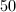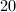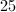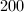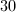Correct answer:Explanation:

We must first start by finding the difference betweenand: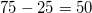Next find the decimal equivalent of%, which is done by dividing by: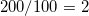Now we divideby.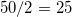Our final answer is, because when it is multiplied by%, it equals the difference betweenand.

### Example Question #11 : Percentages

Solve: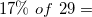Possible Answers: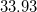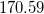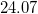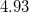Correct answer:Explanation: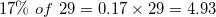### Example Question #2 : Use Proportional Relationships To Solve Multistep Ratio And Percent Problems: Ccss.Math.Content.7.Rp.A.3

What is the sale price of a game if it is% off the original price of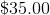?

Possible Answers: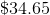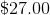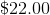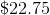Correct answer:Explanation:

First, multiply: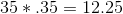Then subtract that amount from the original price: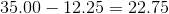Answer: The sale price is.

### Example Question #2 : Use Proportional Relationships To Solve Multistep Ratio And Percent Problems: Ccss.Math.Content.7.Rp.A.3

What is the sale price of a carton of milk if it is% off the original price of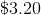?

Possible Answers: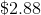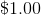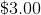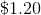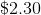Correct answer:Explanation:

First, multiply: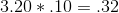Then subtract that amount from the original price: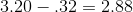Answer: The sale price is.

### Example Question #1 : Use Proportional Relationships To Solve Multistep Ratio And Percent Problems: Ccss.Math.Content.7.Rp.A.3

What is the sale price of a pair of shoes if it is% off the original price of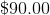?

Possible Answers: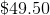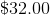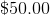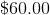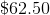Correct answer:Explanation:

First, multiply: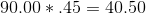Then subtract that amount from the original price: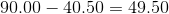Answer: The sale price is.

### Example Question #21 : Percentages

Find the equivalent of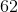%.

Possible Answers: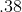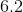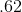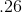Correct answer:Explanation:

Divide: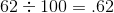Answer:### Example Question #21 : Percentages

Find the equivalent of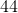%.

Possible Answers: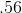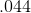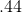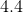Correct answer:Explanation:

Divide: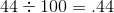Answer:### Example Question #21 : Percentages

Find the equivalent of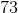%.

Possible Answers: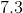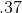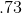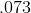Correct answer:Explanation:

Divide: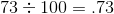Answer:### Example Question #21 : Percentages

Find the equivalent of%.

Possible Answers: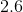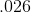Correct answer:Explanation:

Divide: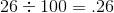Answer:### Example Question #21 : How To Work With Percentages

A sweater costs 100 dollars. It is then discounted 20%. A week later it is discounted another 25%. What is the final price of the sweater?

Possible Answers:

50 dollars

45 dollars

55 dollars

60 dollars

Correct answer:

60 dollars

Explanation:

When the 100 dollar shirt is discounted 20%, it will be discounted 20 dollars, leaving the price at 80 dollars.

When 80 dollars is then discounted another 25%, that value is equal to another 20 dollars.

100 dollars less 20 dollars, less another 20 dollars is 60 dollars.

Thus, 60 dollars is the final price.

### All ISEE Middle Level Math Resources